# List all Worksheet Names

This post will guide you how to get a list of all worksheet names in an excel workbook. How do I List the Sheet names with Formula in Excel. How to generate a list of all sheet tab names using Excel VBA Code.

Assuming that you have a workbook that has hundreds of worksheets and you want to get a list of all the worksheet names in the current workbook. And the below will introduce 3 methods with you.

## Get All Worksheet Names Manually

If there are only few worksheets in your workbook, and you can get a list of all worksheet tab names by manually. Let’s see the below steps:

#2 double click on the sheet’s name in the sheet tab. Press Ctrl + C shortcuts in your keyboard to copy the selected sheet.

#3 create a notepad file, and then press Ctrl +V to paste the sheet name.

#4 follow the above steps 2-3 to copy&paste all worksheet names into notepad file.

## Get All Worksheet Names with Formula

You can also use a formula to get a list of all worksheet names with a formula. You can create a formula based on the LOOKUP function, the CHOOSE function, the INDEX function, the MID function, the FIND function and the ROWS function. Just do the following steps:

#1 go to FORMULAS tab, click Name Manager command under Defined Names group. The Name Manager dialog will open.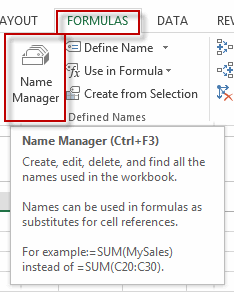#2 click New… button to create a define name, type Sheets in the Name text box, and type the formula into the Refers to text box.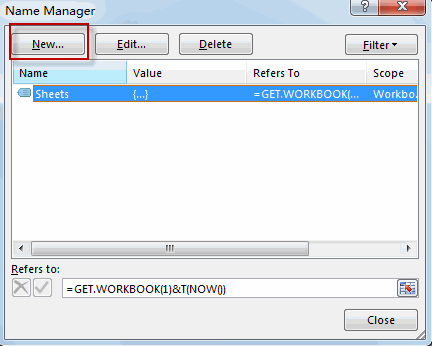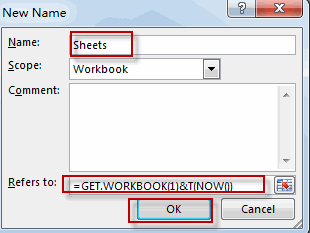`=GET.WORKBOOK(1)&T(NOW())`

#3 Type the following formula into a blank cell and press Enter key in your keyboard, and then drag the autofill handle over others cells to get the rest sheet names.

`=LOOKUP("xxxxx",CHOOSE({1,2},"",INDEX(MID(Sheets,FIND("]",Sheets)+1,255),ROWS(A\$1:A1))))`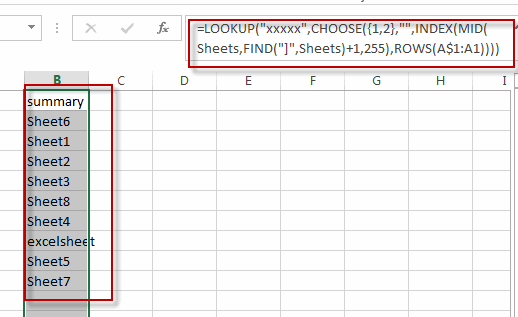You will see that all sheet names have been listed in the cells.

## Get All Sheet Names with Excel VBA Macro

You can also use an Excel VBA Macro to quickly get a list of all worksheet tab names in your workbook. Just do the following steps:

#1 open your excel workbook and then click on “Visual Basic” command under DEVELOPER Tab, or just press “ALT+F11” shortcut.#2 then the “Visual Basic Editor” window will appear.

#3 click “Insert” ->”Module” to create a new module#4 paste the below VBA code into the code window. Then clicking “Save” button.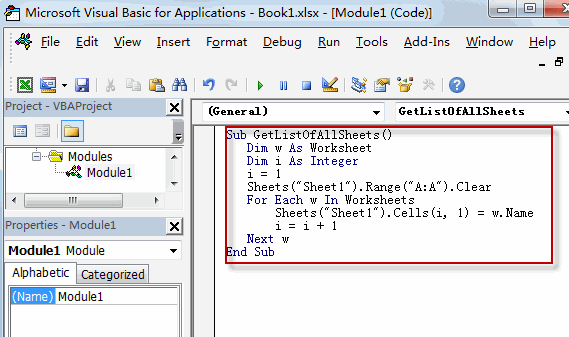```Sub GetListOfAllSheets()
Dim w As Worksheet
Dim i As Integer
i = 1
Sheets("Sheet1").Range("A:A").Clear
For Each w In Worksheets
Sheets("Sheet1").Cells(i, 1) = w.Name
i = i + 1
Next w
End Sub```

#5 back to the current worksheet, then run the above excel macro. Click Run button.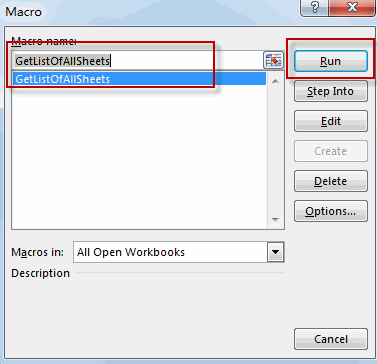#6 Let’s see the result.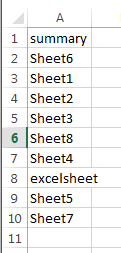### Related Functions

• Excel MID function
The Excel MID function returns a substring from a text string at the position that you specify.The syntax of the MID function is as below:= MID (text, start_num, num_chars)…
• Excel LOOKUP function
The Excel LOOKUP function will search a value in a vector or array.The LOOKUP function is a build-in function in Microsoft Excel and it is categorized as a Lookup and Reference Function.The syntax of the LOOKUP function is as below:= LOOKUP (lookup_value, lookup_vector, [result_vector])…
• Excel Choose Function
The Excel CHOOSE function returns a value from a list of values. The CHOOSE function is a build-in function in Microsoft Excel and it is categorized as a Lookup and Reference Function.The syntax of the CHOOSE function is as below:=CHOOSE (index_num, value1,[value2],…)…
• Excel INDEX function
The Excel INDEX function returns a value from a table based on the index (row number and column number)The INDEX function is a build-in function in Microsoft Excel and it is categorized as a Lookup and Reference Function.The syntax of the INDEX function is as below:= INDEX (array, row_num,[column_num])…
• Excel ROWS function
The Excel ROWS function returns the number of rows in a cell reference.The syntax of the ROWS function is as below:= ROWS(array)…
• Excel FIND function
The Excel FIND function returns the position of the first text string (sub string) within another text string.The syntax of the FIND function is as below:= FIND(find_text, within_text,[start_num])